# Catch up on the latest AI articles# VFS: Effective Rerepsentation For Long-Horizon Tasks With Value Function

3 main points
✔️ Proposal of a representation consisting of value functions of low-level skills, Value Functional Space
✔️ Higher success rate in Model-free RL and Model-Based RL compared to the Baseline method
✔️ Model-free RL experiments show high generalization performance for unknown environments

written by Dhruv ShahPeng XuYao LuTed XiaoAlexander ToshevSergey LevineBrian Ichter
(Submitted on 4 Nov 2021 (v1), last revised 29 Mar 2022 (this version, v2))
Accepted to ICLR 2022
Subjects: Machine Learning (cs.LG); Artificial Intelligence (cs.AI); Robotics (cs.RO)code：The images used in this article are from the paper, the introductory slides, or were created based on them.

## backdrop

Reinforcement Learning (RL) has become capable of learning and solving a variety of complex tasks, but the longer the horizon, the worse the performance. In Hierarchical RL (Hierarchical Reinforcement Learning), for tasks with long horizons, skills with short horizons called low-level skills (e.g., grasping and placing objects in a robot) are abstracted into actions (action abstraction). This Hierarchical RL can also improve the state abstraction itself.

In this paper, we consider that state abstraction is determined by the ability of low-level skill policy to execute skills under the state of the environment. We proposed Value Function Spaces, which use the state abstraction as a state abstraction.

In this paper, we show that the value function spaces improve the performance on long-horizon tasks for both model-free and model-based tasks, and also improve the performance on zero-shot generalization for performance compared to the conventional method.

In the following chapters, we will introduce the detailed methods and experiments.

## technique

The value function in RL can be considered to be very closely related to affordances, and the value function indicates the feasibility of the learned skill. Using this property, a skill-centric representation of the state of the environment, which consists of the skill value function representing the affordances of low-level skills, can be created to perform high-level planning. This is an overview of the main methods in this thesis. The detailed method will be explained throughout this chapter.

The first assumption is that each skill is trained by a sparse reward so that the maximum value of each value is 1. semi-Markov Decision Process (SMDP) $M(S, O, R, P, \tau, \gamma)$ is assumed and If k skills are trained, using the value function $V_{o_{i}}$ for each skill, the skill-centric representation is a k-dimensional representation $Z(s_{t}) = [V_{o_{1}}(s_{t}), V_{o_{2}}(s_{t}),... V_{o_{k}}(s_{t})]$. In this paper, we call this representation Value Function Spaces (VFS).

The following figure shows the abstraction of state in the desk rearrangement task. First, an observation, such as an image, is abstracted into an 8-dimensional value function tuple consisting of 8 skills. This representation also holds information about the location, for example, if a drawer is closed, the value of the corresponding skill is 1 because the task is completed. In addition, it also holds information about the preconditions for the interaction (for example, a high value for the "Pick" skill means that two blocks are ready to be lifted), and the effect of executing a feasible skill (the "Open drawer" skill has a value of $t_{1 }$ and is therefore very suitable for high-level planning.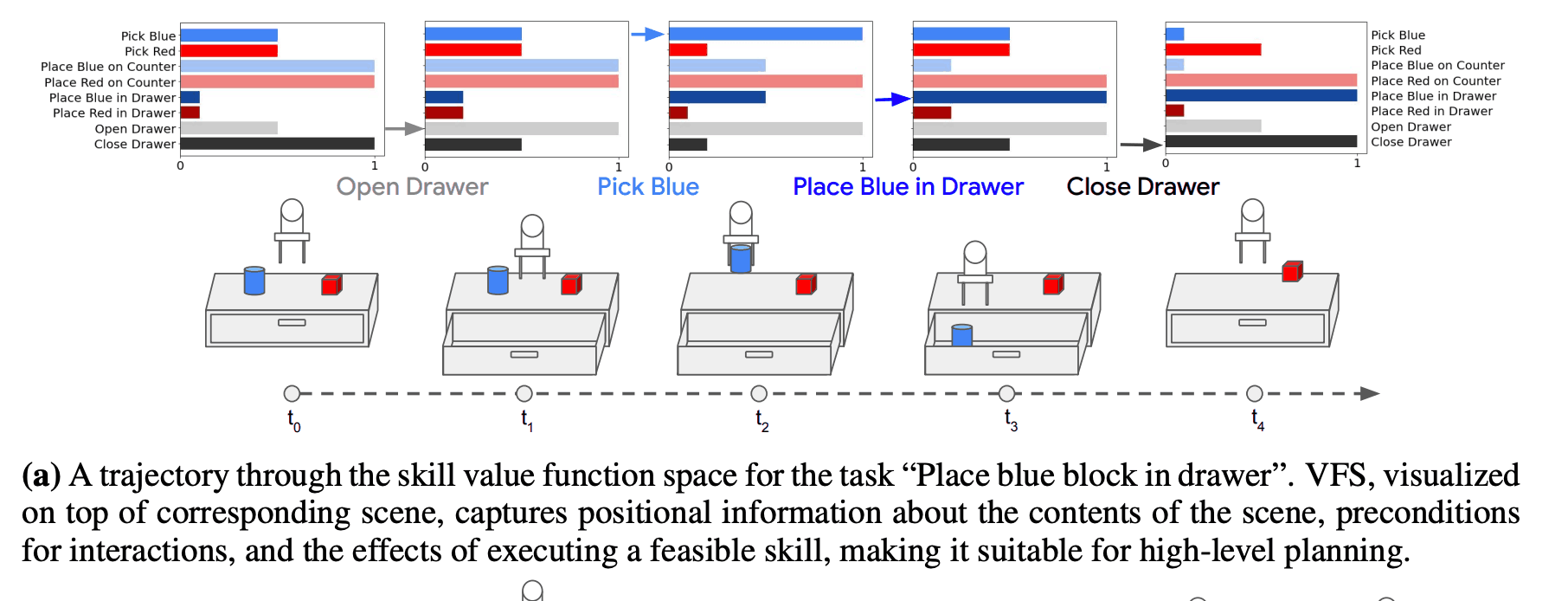In addition, because VFS is a skill-centric representation, it is robust to background distractors and task-irrelevant information in a hypothetical environment. Therefore, it is thought that VFS can adapt to unknown environments, and we evaluated its effectiveness in this paper. The figure below shows an example of a desk rearrangement task, which is different in terms of image information, but similar in terms of VFS, and these states can be considered "functionally equivalent". In other words, no matter where the red cube is in the drawer and the blue cube is on the desk, they are all in the same state from the skill point of view.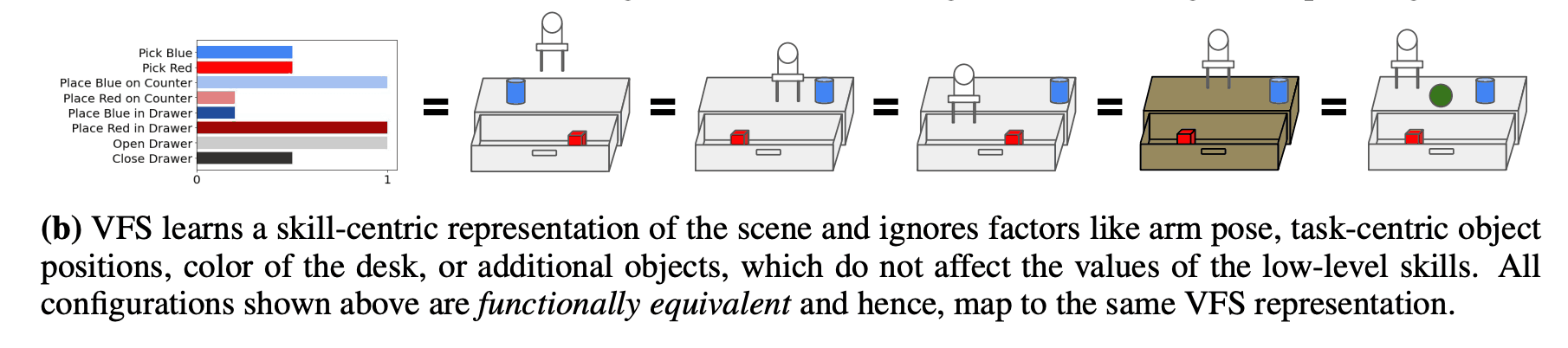In this study, we evaluated VFS in both mode-free and model-based RL. In the following sections, we introduce how VFS can be used in both model-free and model-based RLs, and the results of our experiments.

## Model-Free RL + VFS

In model-free RL, we used Hierarchical RL, where VFS is used as the observation of high-level policy, and each pre-trained skill is used as the low-level policy. In our experiments, we compared the performance of the long-horizon task.

The high-level policy can be obtained by learning a Q-function $Q(Z, o)$ using DQN and setting $\pi_{Q}(Z) = argmax_{o_{i}} Q(Z, o_{o})$. In this training, a mini-batch $(Z_{t}, o_{t}, r_{t}, Z_{t+1})$ of VFS is sampled from the replay buffer and the Q-function is obtained by optimizing the following error function.

$$L=\mathbb{E} (Q(Z_{t}, o_{t}) - y_{t})^{2}$$

where $y_{t}=r_{t} + \gamma max_{o_{t'}}Q(Z_{t+1}, o_{t'})$. However, in this paper we actually used a derived algorithm called DDQN to further stabilize the learning.

To compare VFS with different representation learning methods concerning the performance of the long-horizon task, this paper presents two tasks in a MiniGrid environment, the
- MultiRoom: a task to solve a maze consisting of up to 10 rooms of different sizes to reach a goal
- KeyCorridor: a task in which the agent must search for a key to open a locked door to reach a goal at the end of up to seven rooms
We evaluated the results concerning the Both tasks are sparse and only reward the player for reaching the goal.

The low-level skills for these tasks are given as GoToObject, PickupObject, DropObject, and UnlockDoor.

The following methods were used as Baseline methods

- Raw Observations
- Autoencoder (AE)
- Contrastive Predicting Coding (CPC)
- Online Variational Autoencoder
- Contrastive Unsupervised Representations for RL (CURL)

The table below shows the results of the experiment. First of all, when learning a high-level policy from raw observations, the success rate is $64^%$ when the number of rooms is two, but the success rate decreases significantly as the number of rooms increases, especially when the number of rooms is ten. The success rate was as low as $29\%$. The performance of AE and CPC also shows an improved success rate compared to the case of the raw observation, but still shows a low success rate since the representation is trained independently of the high-level policy objective function. In the case of VAE and CURL, the success rate is higher than that of AE and CPC because they are trained at the same time as the objective function of the high-level policy, but the success rate is lower because the learned representation has a less direct impact on the task. The following is an example of the use of the In contrast, VFS outperforms the baseline method in most tasks, and this is because it maximizes the direct impact of the representation on the task by clearly capturing the ability to execute low-level policy skills. The reason for this is that we were able to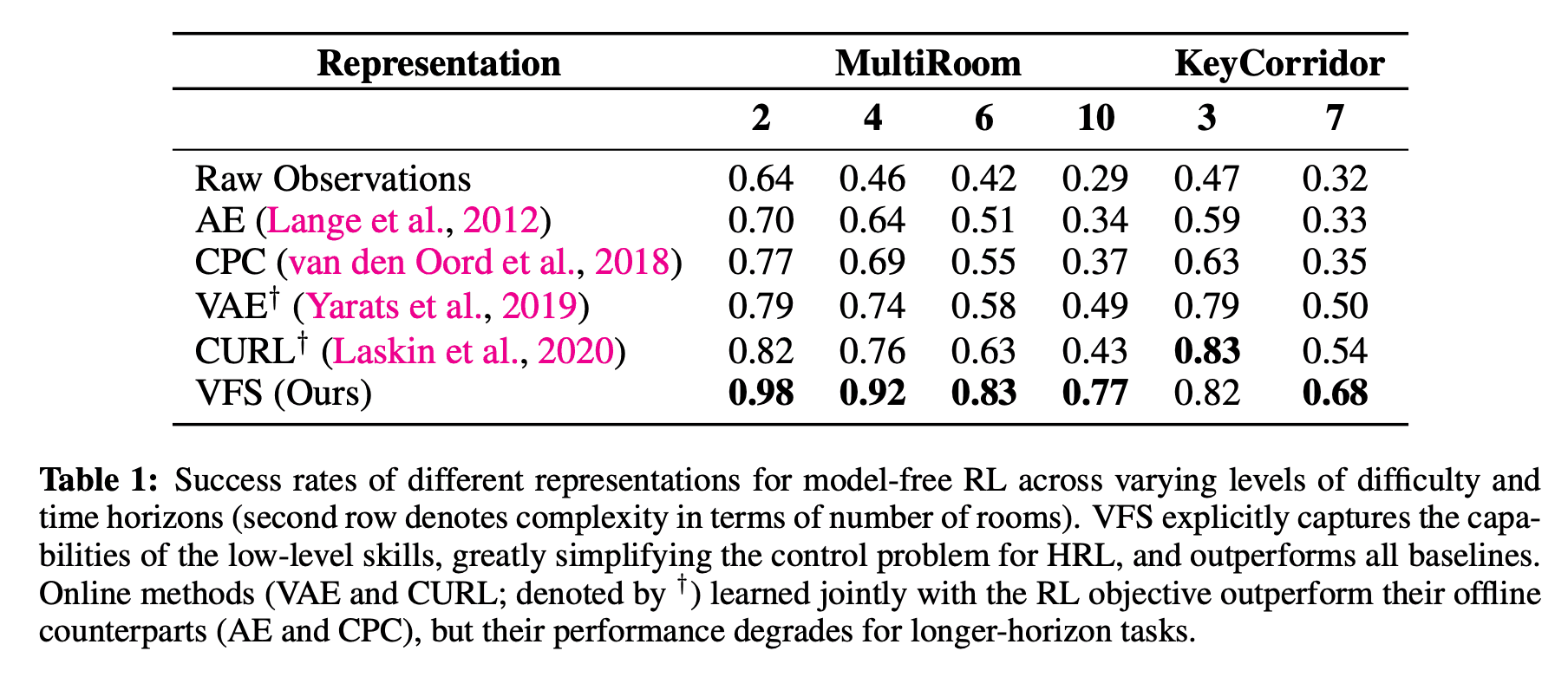The figure below shows the trajectory of successfully solving the task using VFS.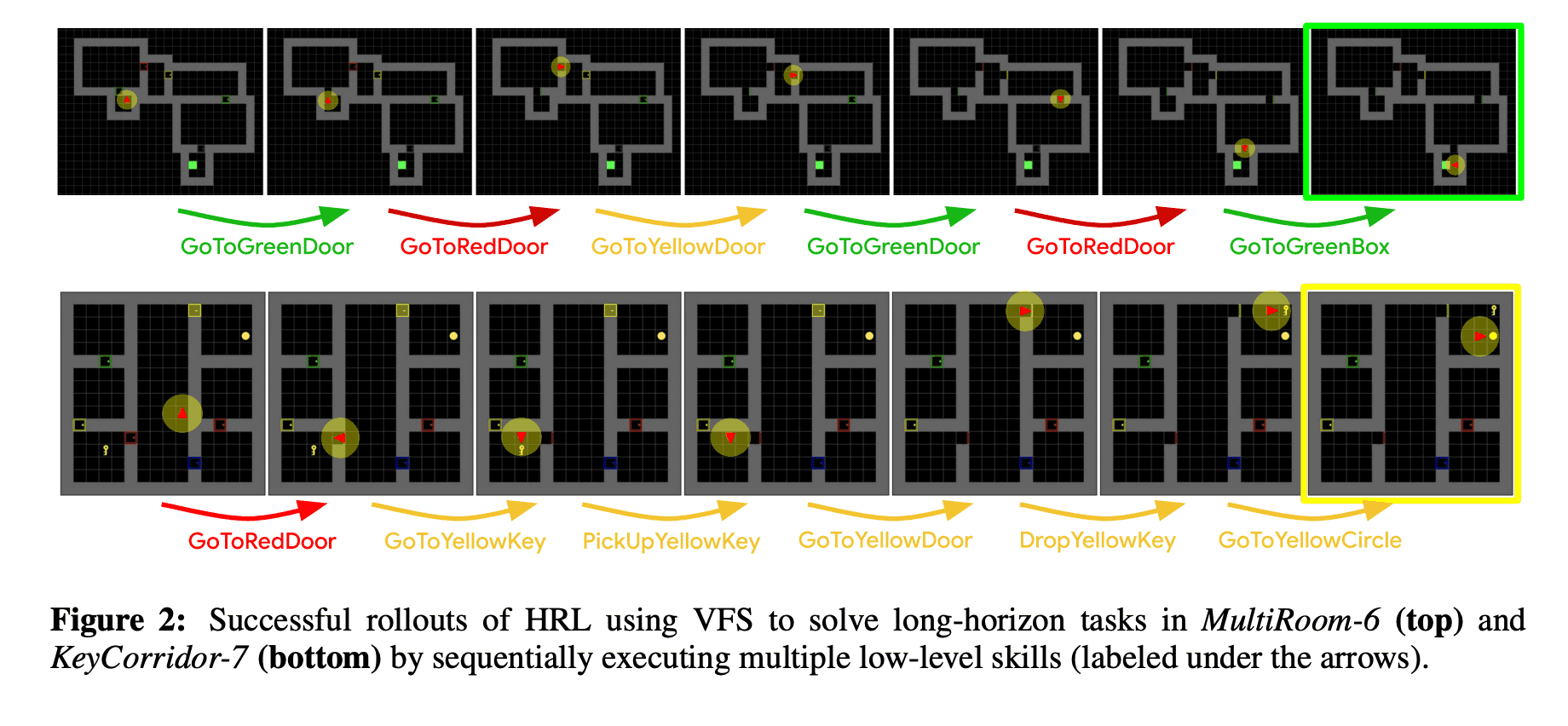### Generalization performance for unknown environments

Finally in this chapter, we evaluated the effectiveness of VFS for unknown environments. In this experiment, as shown in the left figure below, we executed the policy learned by KeyCorridor in MultiRoom and evaluated how much success rate it shows. The results are summarized in the table on the right, which shows that AP and CPC show a success rate of $47%$ in MR4, while MR10 shows an even lower success rate of $20%$. The reason for this is that the representation was learned at the same time and overfitted to the KeyCorridor environment. In contrast, VFS succeeds in zero-shot generalization and shows higher success rates than the other methods: $87^%$ for MR4 and $67^%$ for MR10. These success rates are close to the performance of the trained baseline, HRL-target, in the MultiRoom environment.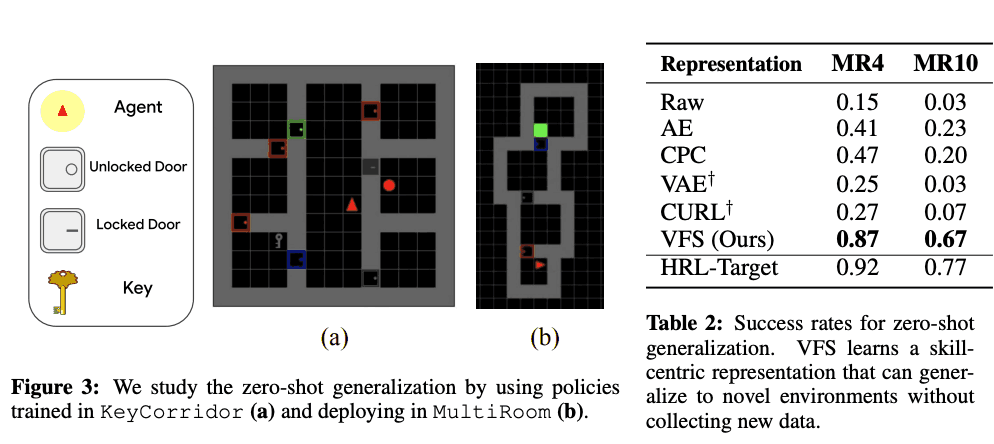## Model-based RL + VFS

In this chapter, we introduce an application to model-based RL that uses VFS as a state for planning (VFS-MB). In this paper, a one-step predictive $Z_{t+1} = \hat{f}(Z_{t}, o_{t})$ is trained using supervised learning. Then, to solve the task, the optimal sequence of skills $(o_{t}, ..., o_{t}, ..., o_{t}, ..., o_{t}, ..., o_{t}, ..., o_{t}, ..., o_{t}) is calculated using$Z_{g}$, the goal representation of the task, and$Cost function $\epsilon$. The following optimization problem is solved to find the optimal skill sequence $(o_{t+H-1})$ using $Z_{g}$, the goal representation of the task, and $\epsilon$, the cost function.

$$(o_{t}, ... ,o_{t+H-1}) = argmin_{o_{t}, ... ,o_{t+H-1}} \epsilon(\hat{Z}_{t+H}, Z_{g}) : \hat{Z_{t}} = Z_{t}, \hat{Z}_{t'+1} = \hat{f}(\hat{Z}_{t'}, o_{t'})$$

And for planning, this paper solves the above optimization problem using the sampling-based method. Specifically, we randomly generated k candidate option sequences $(o_{t}, o_{t+1},...)$ and predict the corresponding $Z$ sequences using the learned $\hat{f}$. Then, we select the one closest to the goal among k candidates and execute the policy using model-predictive control. In this case, only the first skill $O_{T}$ of the generated sequence is executed. The following figure shows these summary diagrams.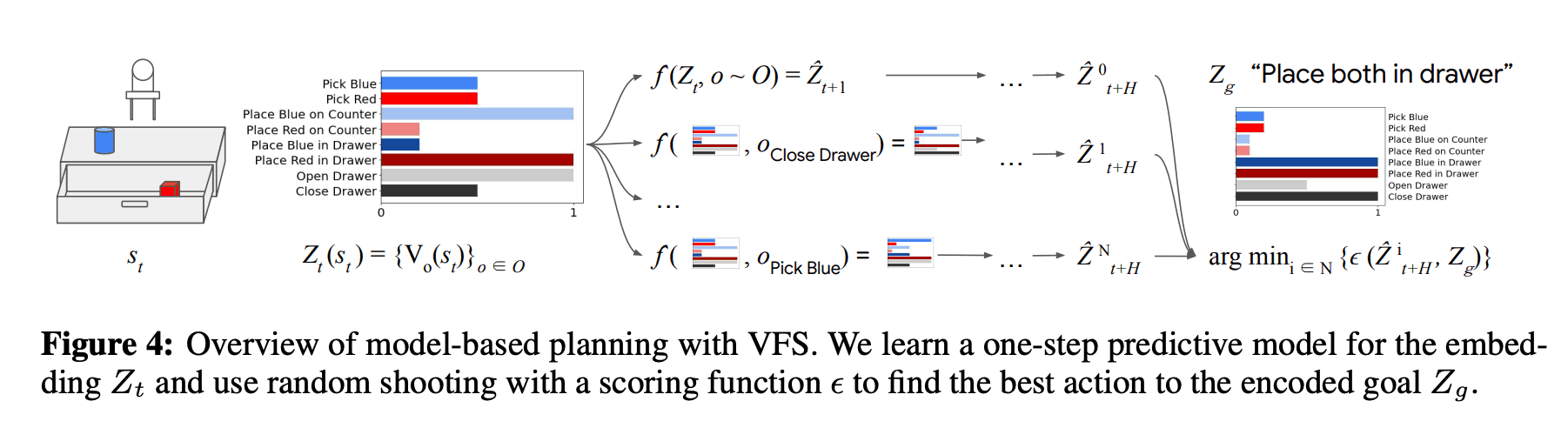### assess

In this paper, we evaluate the 8DoF robot in the object rearrangement task as follows: As in the Model-free RL experiment, we assume that the low-level policy has been learned in advance. skill, that is, the skill to move object A close to object B. In this experiment, there are 10 objects and 9 objects to move to, so a total of 90 skills are used. In addition, only the image information as shown in the figure below is given as the observation of the policy.

VAE and CPC were used as baseline methods, and each method was evaluated 20 times. As shown in the table below, VFS has a higher success rate than the other methods, which indicates that VFS can provide an effective representation that includes information and skill affordances of the scene. We also found that the success rate is similar to that of the oracle baseline when the low-dimensional state is used as input instead of receiving image information as input.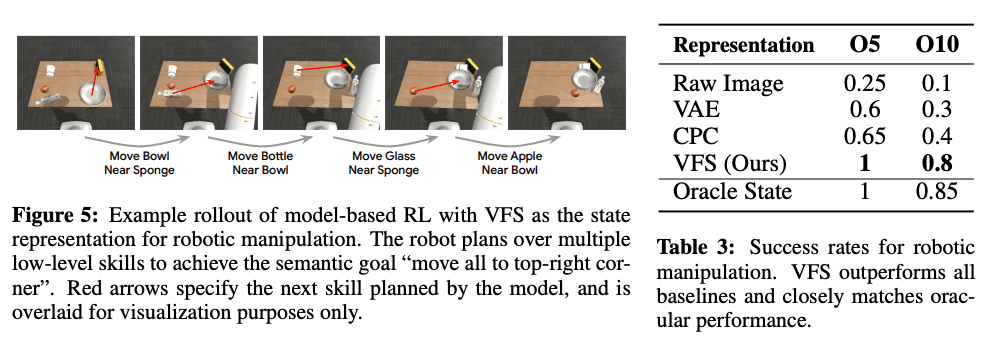Finally, to investigate what elements are captured by the VFS, we visualized the representation using t-SNE as shown in the figure below. The different color ranges in the figure below represent different objects being grabbed by the robot arm with the chocolate being on the table. These indicate that VFS is successfully grabbing information about the object.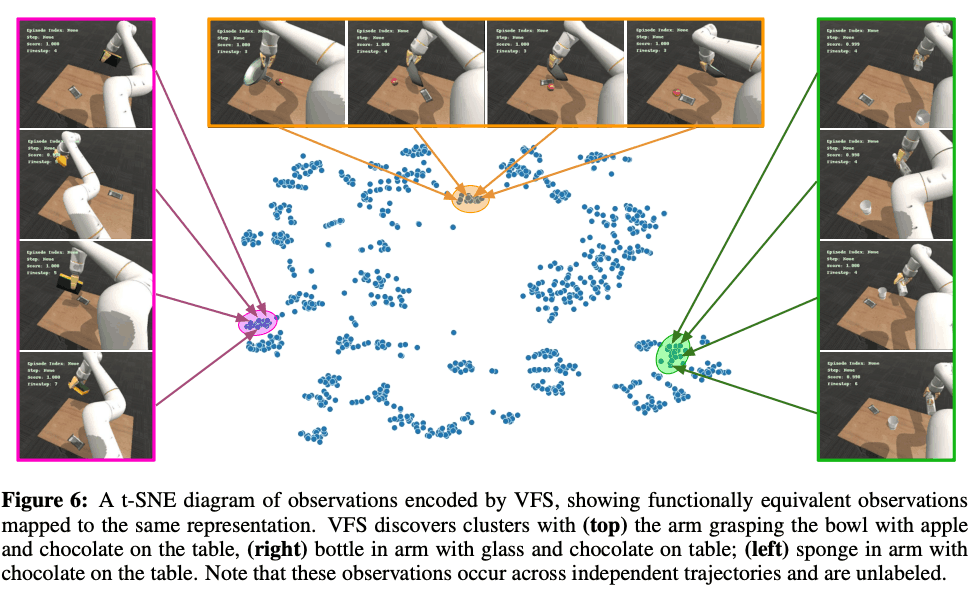## summary

In this article, we introduced a very simple method to improve the success rate of long-horizon tasks and generalization performance to unknown environments by using the value function of low-level skill as a representation. The long-horizon task can be used Since it is a common task in our daily life, we expect that more papers on long-horizon tasks will be published in the future due to its importance.

If you have any suggestions for improvement of the content of the article,